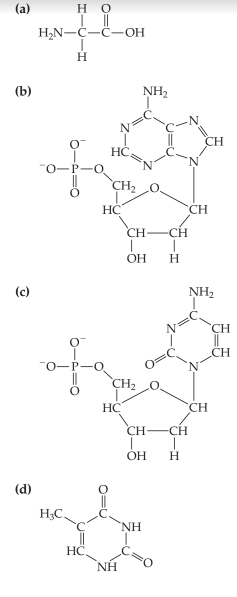×
Get Full Access to Introductory Chemistry - 5 Edition - Chapter 19 - Problem 70p
Get Full Access to Introductory Chemistry - 5 Edition - Chapter 19 - Problem 70p

×

# Solved: Determine whether each structure is that of a nucleotide. For those that areISBN: 9780321910295 34

## Solution for problem 70P Chapter 19

Introductory Chemistry | 5th Edition

• Textbook Solutions
• 2901 Step-by-step solutions solved by professors and subject experts
• Get 24/7 help from StudySoup virtual teaching assistantsIntroductory Chemistry | 5th Edition

4 5 1 285 Reviews
14
4
Problem 70P

Determine whether each structure is that of a nucleotide. For those that are nucleotides, identify the base as A, T, C, or G.Step-by-Step Solution:

Solution 70P :

Step 1:

Nucleotides are the individual units that compose the nucleic acids. Nucleotides consists of 3 parts : (1) A phosphate, (2) a sugar and (3) a base.

Each base in the nucleotide is complementary which is capable of pairing precisely. with only one other base. Base Adenine (A), hydrogen-bonds only with the base thymine (T). And base cytosine (C) hydrogen-bonds only with base guanine (G).

Step 2 of 2

##### ISBN: 9780321910295

Unlock Textbook Solution